The Einstein velocity relationship transforms a measured velocity as seen in one inertial frame of reference (u) to the velocity as measured in a frame moving at velocity v with respect to it (u'). In problems involving more than two objects, the main difficulty is the assignment of velocity to all the objects.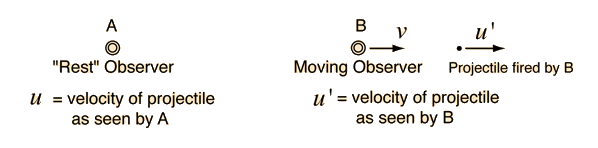If A sees B moving at velocity v, then a velocity measured by B (u') would be seen by A as: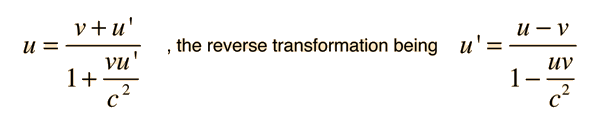These relationships make perfect sense at low speeds where both denominators approach 1.

Index

 HyperPhysics***** Relativity R Nave
Go Back

# Relativistic Relative Velocity

The speed of light is the speed limit of the universe, so it follows that no observer will see any other observer approaching or receding at a speed greater than c. But what if observers A and B are both moving toward each other with speeds approaching c as seen by an external observer? How will A and B measure their relative speeds? This is an example of Einstein velocity addition. In the calculation below, velocities to the right are taken as positive.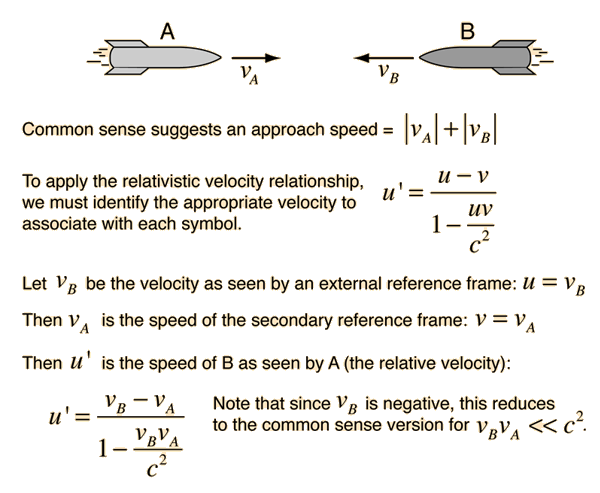If vA = c and vB = c then u' = c.

Exactly the same magnitude of velocity is obtained if you evaluate the approach velocity as observed by B.
Index

 HyperPhysics***** Relativity R Nave
Go Back

# Relativistic Projectile

Now if spacecraft A fires a projectile at velocity Vp as measured in A's frame of reference, then the two relevant questions are:

1. How fast will an external observer see the projectile traveling?

2. How fast will B see the projectile approaching?

This is an example of Einstein velocity addition.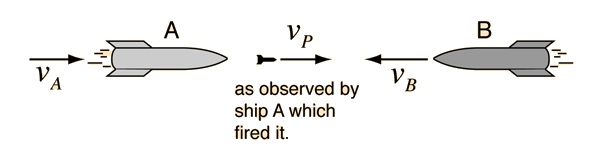Taking question #1 first, then in the standard form: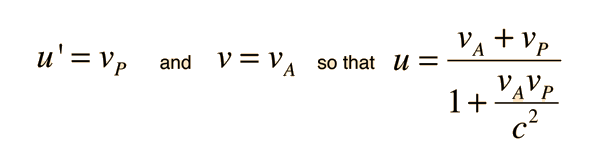If vA = c and vP = c then u = c.

Note that for low velocities, this is the simple sum of the velocities of the firing spacecraft and the projectile as seen by that spacecraft.

Now to calculate the approach velocity as seen by the targeted spacecraft B: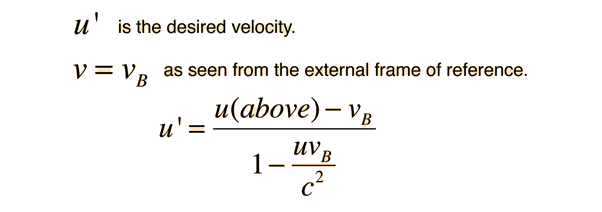If u(above) = c and vB = c then u' = c.

For low speeds, this expression yields a value equal to the sum of the magnitudes of the three velocities as indicated in the diagram. The Einstein velocity addition gaurantees that the resultant velocity does not exceed c, even if all three of the indicated velocities approach c!
Index

 HyperPhysics***** Relativity R Nave
Go Back